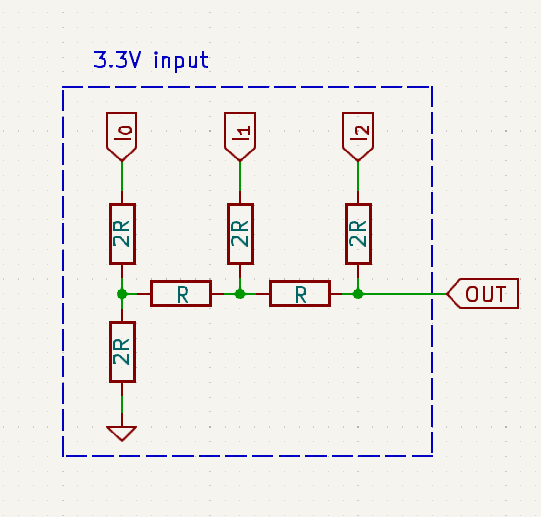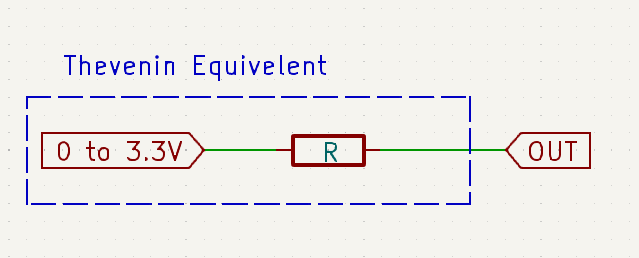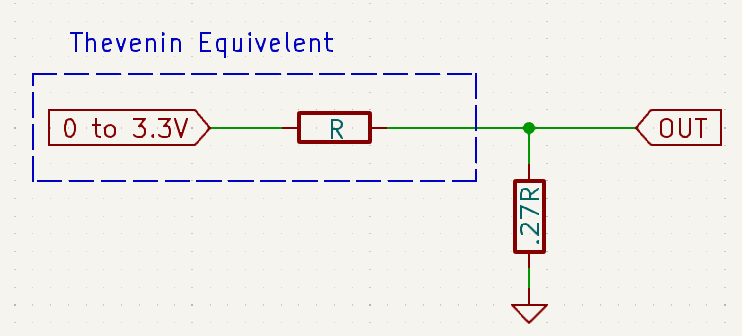# The Design Process

VGA needs an analog signal, while one can use a DAC chip for each color, it would be much simpler (and cheaper) to just use an R2R ladder.

## R2R

Not much to say about it, a simple R2R would nicely convert Digital values to Analog, I have decied to use 3 bits for each color, for a total of $$2^3 = 8$$ levels of a color. Since VGA uses 3 different colors, I get a total of $$8\times 3 = 24$$ colors, which isn’t that bad.## Voltage Level

VGA uses $$0.7V$$, while my FPGA has a logic output of 3.3V, this could easily be solved using a voltage divider.Luckliy, The thevenin equivalent circuit of R2R ladder can easily be found, then one can construct a voltage divider to achieve a value of $$0.7V$$ (or lower).

$3.3V \times \frac{x}{x + R} \le 0.7$

Solving for $$x$$, we see that $$x \le 0.27R$$.## Choosing Values for R

Now I know the I need the following values, $$R$$, $$2R$$ and less than $$0.27R$$. I will spare you my trail-and-error, I found that $$5K\Omega$$ seems like a good choice. The nearest values I had with me are $$5.1K\Omega$$, $$10K\Omega$$ and $$1K\Omega$$.